# Java内存结构

Java虚拟机要运行程序，必须要对内存进行空间的分配和管理。

Java运行程序，会从OS来申请内存的。并不是将整个内存都给Java来使用。

Java内存主要分为5部分，目的是更加方便的管理。

## JVM内存划分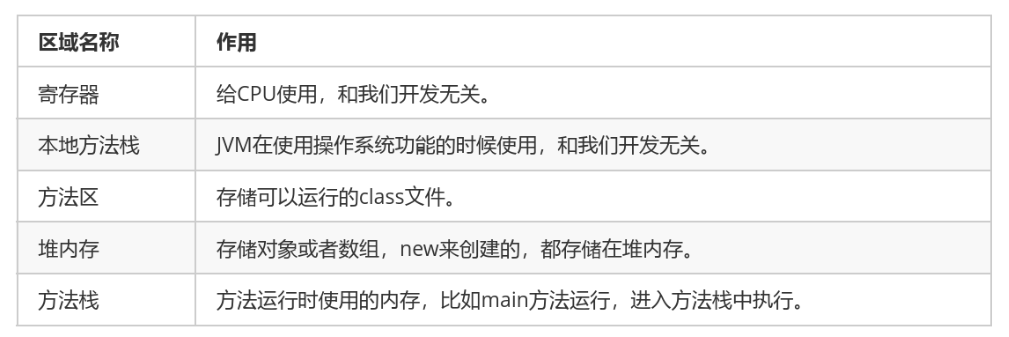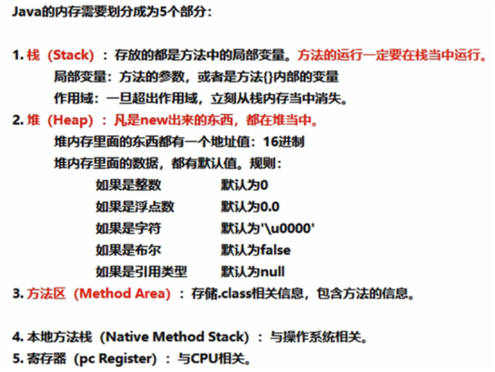# 数组定义

## 方式一 动态初始化方式

`````` 数组存储的数据类型[] 数组名字 = new 数组存储的数据类型[长度];
``````

[] : 表示数组。

new：关键字，创建数组使用的关键字。

[长度]：数组的长度，表示数组容器中可以存储多少个元素。

``````int[] arr = new int;
int arr[] = new int;//类似c中的写法
``````

## 方式二 静态初始化方式

``````数据类型[] 数组名 = new 数据类型[]{元素1,元素2,元素3...};
``````

``````int[] arr = new int[]{1,2,3,4,5};
``````

## 方式三 静态初始化方式-简化版

``````数据类型[] 数组名 = {元素1,元素2,元素3...};
``````

``````int[] arr = {1,2,3,4,5};
``````

# 数组访问

## 索引

``````数组名[索引]
``````

## 长度属性

``````public static void main(String[] args) {
int[] arr = new int[]{1,2,3,4,5};
//打印数组的属性，输出结果是5
System.out.println(arr.length);
System.out.println(arr);
System.out.println(arr);
System.out.println(arr);
System.out.println(arr);
System.out.println(arr);
}
``````

``````public static void main(String[] args) {
//定义存储int类型数组，赋值元素1，2，3，4，5
int[] arr = {1,2,3,4,5};
//为0索引元素赋值为6
arr = 6;
//获取数组0索引上的元素
int i = arr;
System.out.println(i);
//直接输出数组0索引元素
System.out.println(arr);
}
``````

# 数组内存结构图

``````public static void main(String[] args) {
int[] arr = new int;
System.out.println(arr);//[I@5f150435
}
``````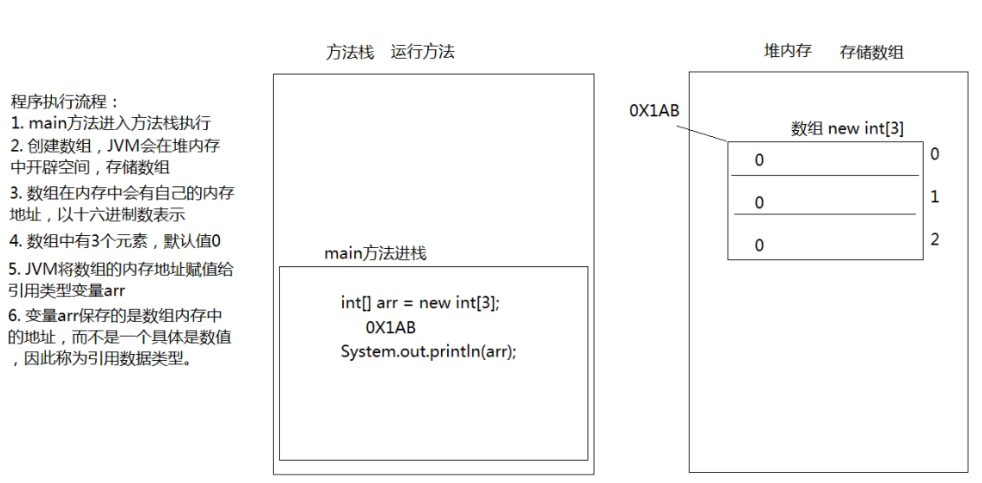``````public static void main(String[] args) {
int[] arr = new int;
int[] arr2 = new int;
System.out.println(arr);
System.out.println(arr2);
}
``````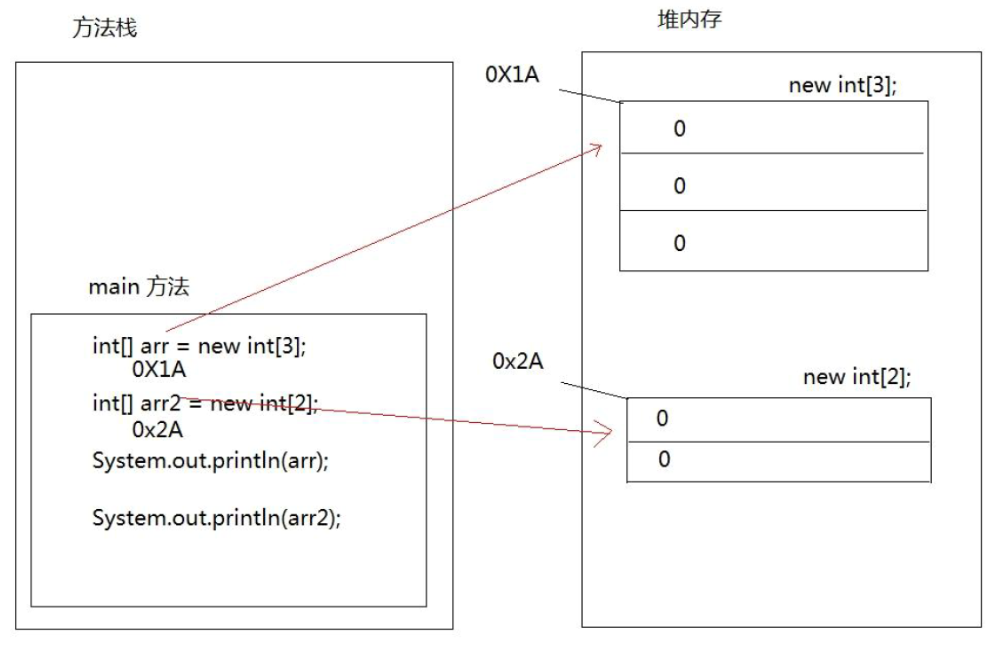``````public static void main(String[] args) {
// 定义数组，存储3个元素
int[] arr = new int;
//数组索引进行赋值
arr = 5;
arr = 6;
arr = 7;
//输出3个索引上的元素值
System.out.println(arr);
System.out.println(arr);
System.out.println(arr);
//定义数组变量arr2，将arr的地址赋值给arr2
int[] arr2 = arr;
arr2 = 9;
System.out.println(arr);
}
``````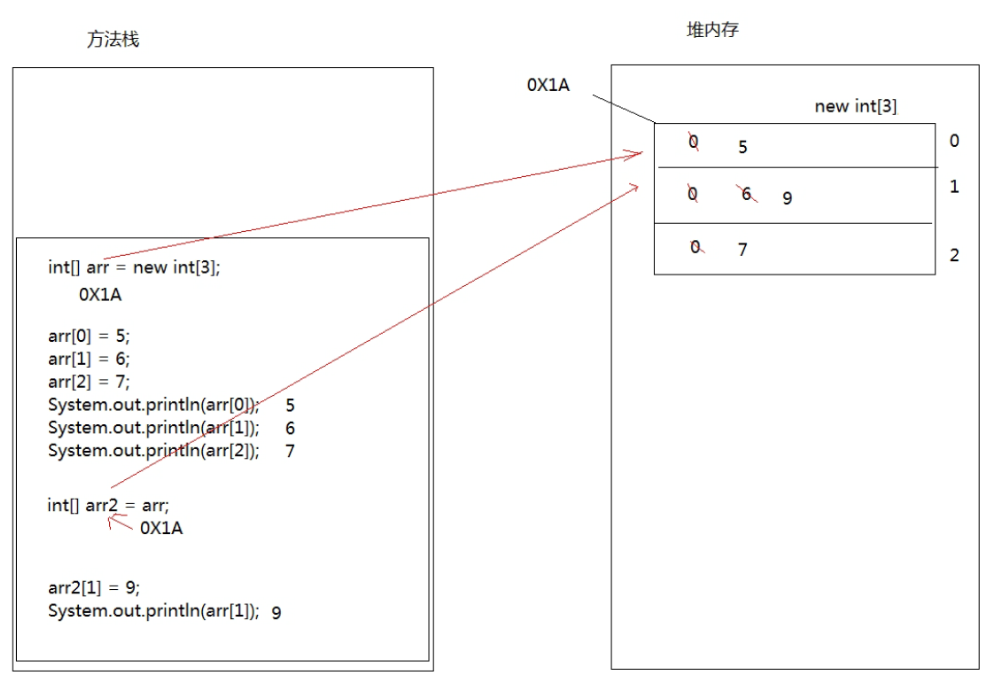# 数组的遍历

``````public static void main(String[] args) {
int[] arr = { 1, 2, 3, 4, 5 };
System.out.println(arr);
System.out.println(arr);
System.out.println(arr);
System.out.println(arr);
System.out.println(arr);
}
``````

``````public static void main(String[] args) {
int[] arr = { 1, 2, 3, 4, 5 };
for (int i = 0; i < arr.length; i++) {
System.out.println(arr[i]);
}
}
``````

``````public static void main(String[] args) {
int[] arr = { 5, 15, 2000, 10000, 100, 4000 };
//定义变量，保存数组中0索引的元素
int max = arr;
//遍历数组，取出每个元素
for (int i = 0; i < arr.length; i++) {
//遍历到的元素和变量max比较
//如果数组元素大于max
if (arr[i] > max) {
//max记录住大值
max = arr[i];
}
}
System.out.println("数组最大值是： " + max);
}
``````

``````public static void main(String[] args) {
int[] arr = { 1, 2, 3, 4, 5 };
/*
循环中定义变量min=0最小索引
max=arr.length‐1最大索引
min++,max‐‐
*/
for (int min = 0, max = arr.length ‐ 1; min <= max; min++, max‐‐) {
//利用第三方变量完成数组中的元素交换
int temp = arr[min];
arr[min] = arr[max];
arr[max] = temp;
}
// 反转后，遍历数组
for (int i = 0; i < arr.length; i++) {
System.out.println(arr[i]);
}
}
``````

# 数组相关异常

## 数组越界异常

``````public static void main(String[] args) {
int[] arr = {1,2,3};
System.out.println(arr);
}
``````

## 数组空指针异常

``````public static void main(String[] args) {
int[] arr = {1,2,3};
arr = null;
System.out.println(arr);
｝
``````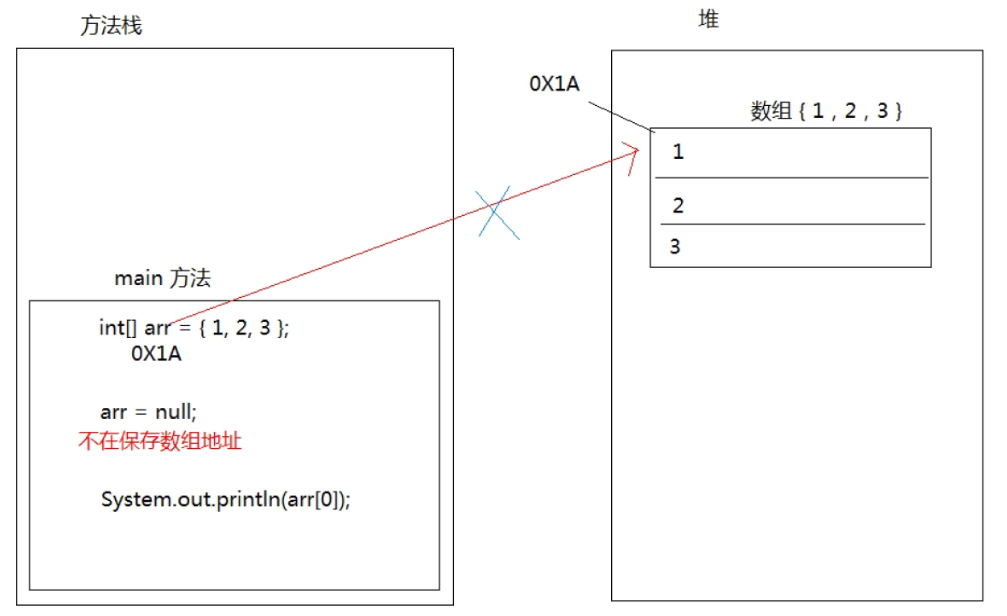# 数组作为方法参数和返回值

## 数组作为方法参数

``````public static void main(String[] args) {
int[] arr = { 1, 3, 5, 7, 9 };
//调用方法，传递数组
printArray(arr);
}
/*
创建方法，方法接收数组类型的参数
进行数组的遍历
*/
public static void printArray(int[] arr) {
for (int i = 0; i < arr.length; i++) {
System.out.println(arr[i]);
}
}
``````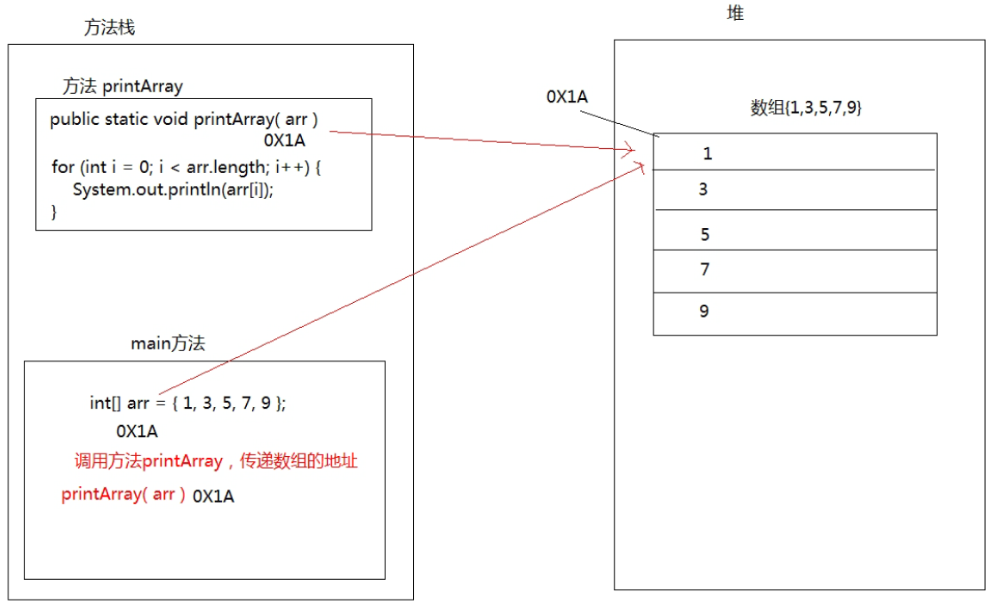## 数组作为方法返回值

``````public static void main(String[] args) {
//调用方法，接收数组的返回值
//接收到的是数组的内存地址
int[] arr = getArray();
for (int i = 0; i < arr.length; i++) {
System.out.println(arr[i]);
}
}
/*
创建方法，返回值是数组类型
return返回数组的地址
*/
public static int[] getArray() {
int[] arr = { 1, 3, 5, 7, 9 };
//返回数组的地址，返回到调用者
return arr;
}
``````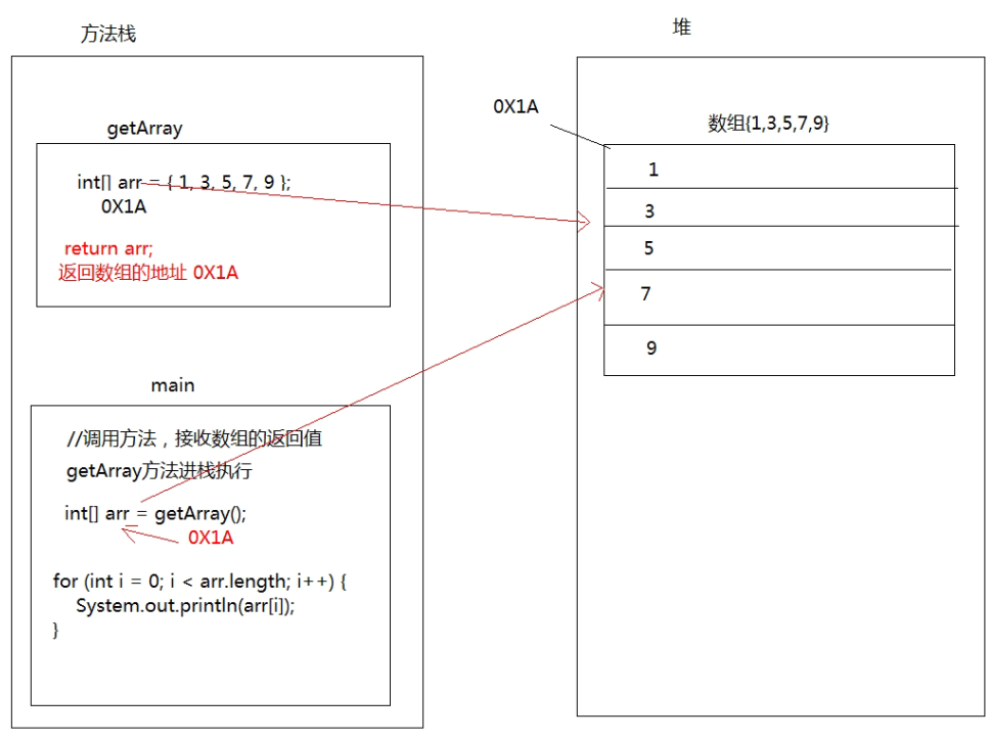## 方法传递参数时参数类型区别

``````public static void main(String[] args) {
int a = 1;
int b = 2;
System.out.println(a);
System.out.println(b);
change(a, b);
System.out.println(a);
System.out.println(b);
}
public static void change(int a, int b) {
a = a + b;
b = b + a;
}
``````
``````public static void main(String[] args) {
int[] arr = {1,3,5};
System.out.println(arr);
change(arr);
System.out.println(arr);
}

public static void change(int[] arr) {
arr = 200;
}
``````

09-内存与数组

(0)
(0)